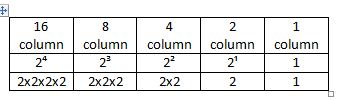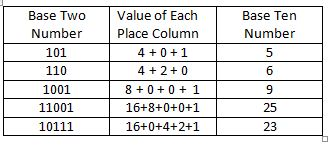# Binary Numbers: Lesson for Kids

Instructor: Suzanne Rose

Suzanne has taught all levels PK-graduate school and has a PhD in Instructional Systems Design. She currently teachers literacy courses to preservice and inservice teachers.

How would you like to learn to 'talk' to a computer? Computers use binary coding to work. In this lesson, you'll learn all about binary numbers, how to read them, and how we use them. Read on to learn 'computer talk!'

## Base Ten and Place Value

Our decimal number system is based on ten, sometimes called base ten. This means that in our number system, we represent amounts using ten numerals: 0, 1, 2, 3, 4, 5, 6, 7, 8, and 9. When we need to write a larger number, we reuse the same numerals and make use of place value.

Place value means that each column, or place, in a number has a particular value. Each place to the left increases by a power of ten. Base ten seems simple and easy to us because we've used it a long time.

## Binary Numbers

The binary number system, also called base two, works similarly to the base ten system. Since it is base two, we can only use two numerals: 0 and 1. With base two, we also use place value: each place to the left increases in size by a factor of 2.

Here are the place values for the first five base two columns.## Changing Base Two to Base Ten

Using the chart that shows the place value for the first five columns in base two, you can convert base two numbers to base ten numbers. Let's do an example together.

You want to change the base two number 101 into a decimal number.

1. To do this, start with the column on the far right, the 1 column. There is a 1 in that column. So you would multiply 1 x 1 = 1.
2. Move to the next column to left, which is the 2 column. You have 0 in that column, so you would multiply 0 x 2 = 0.
3. Then move to the next column to left, which is the 4 column. You have a 1 in that column, so you would multiply 1 x 4 = 4.
4. Add the numbers you have gotten together to get the base ten number. 1 + 0 + 4 = 5. So, 101 in base two (binary) is the same as the number 5 in base ten (decimal).

Here are a few more examples of base two numbers changed into base ten numbers:## Origins of Binary Numbers

Aboriginal peoples in Australia used a base two counting system, so binary numbers are not new. African tribes used a binary system of high and low drum beats to send messages to people at a distance. In 1666, in a published article, Gottfried Leibniz explained how thought could be represented by patterns of numbers, which set the stage for the use of binary numbers in later machines.

To unlock this lesson you must be a Study.com Member.

### Register to view this lesson

Are you a student or a teacher?

#### See for yourself why 30 million people use Study.com

##### Become a Study.com member and start learning now.
Back
What teachers are saying about Study.com

### Earning College Credit

Did you know… We have over 220 college courses that prepare you to earn credit by exam that is accepted by over 1,500 colleges and universities. You can test out of the first two years of college and save thousands off your degree. Anyone can earn credit-by-exam regardless of age or education level.